### Home > AC > Chapter 14 > Lesson 14.2.1.4 > Problem2-59

2-59.
1. A dart hits each dartboard below at random. What is the probability that the dart will land in the darkly shaded area? Homework Help ✎

1.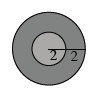2.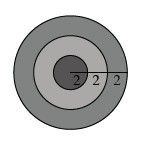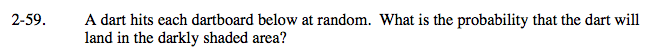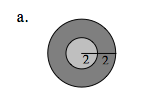First find the total area of the dartboard. A = r2?

16π

Subtract the area of the lighter, smaller circle from the total area of the whole circle.

16π − 4π = 12π

Divide the difference between the areas of the dartboardand the small circle by the area of the dartboard.

$\frac{12\pi}{16\pi}$

$\text{The probability is }\frac{3}{4}.$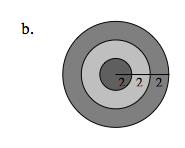Use the same method from part (a).